# MLP, Numpy, TF2 – performance issues – Step III – a correction to BW propagation

In the last articles of this series

we looked at the FW-propagation of the MLP code which I discussed in another article series. We found that the treatment of bias neurons in the input layer was technically inefficient due to a collision of C- and F-contiguous arrays. By circumventing the problem we could accelerate the FW-propagation of big batches (as the complete training or test data set) by more than a factor of 2.

In this article I want to turn to the BW propagation and do some analysis regarding CPU consumption there. We will find a simple (and stupid) calculation step there which we shall replace. This will give us another 15% to 22% performance improvement in comparison to what we have reached in the last article for MNIST data:

• 9.6 secs for 35 epochs and a batch-size of 500
• and 8.7 secs for a batch-size of 20000.

# Present CPU time relation between the FW- and the BW-propagation

The central training of mini-batches is performed by the method "_handle_mini_batch()".

```#
''' -- Method to deal with a batch -- '''
def _handle_mini_batch (self, num_batch = 0, num_epoch = 0, b_print_y_vals = False, b_print = False, b_keep_bw_matrices = True):
''' .... '''
# Layer-related lists to be filled with 2-dim Numpy matrices during FW propagation
# ********************************************************************************
li_Z_in_layer  = [None] * self._n_total_layers # List of matrices with z-input values for each layer; filled during FW-propagation
li_A_out_layer = li_Z_in_layer.copy()          # List of matrices with results of activation/output-functions for each layer; filled during FW-propagation
li_delta_out   = li_Z_in_layer.copy()          # Matrix with out_delta-values at the outermost layer
li_delta_layer = li_Z_in_layer.copy()          # List of the matrices for the BW propagated delta values
li_D_layer     = li_Z_in_layer.copy()          # List of the derivative matrices D containing partial derivatives of the activation/ouput functions
li_grad_layer  = li_Z_in_layer.copy()          # List of the matrices with gradient values for weight corrections

# Major steps for the mini-batch during one epoch iteration
# **********************************************************

#ts=time.perf_counter()
# Step 0: List of indices for data records in the present mini-batch
# ******
ay_idx_batch = self._ay_mini_batches[num_batch]

# Step 1: Special preparation of the Z-input to the MLP's input Layer L0
# ******
# ts=time.perf_counter()
# slicing
li_Z_in_layer = self._X_train[ay_idx_batch] # numpy arrays can be indexed by an array of integers

# transposition
#~~~~~~~~~~~~~~
li_Z_in_layer = li_Z_in_layer.T
#te=time.perf_counter(); t_batch = te - ts;
#print("\nti - transposed inputbatch =", t_batch)

# Step 2: Call forward propagation method for the present mini-batch of training records
# *******
#tsa = time.perf_counter()
self._fw_propagation(li_Z_in = li_Z_in_layer, li_A_out = li_A_out_layer)
#tea = time.perf_counter(); ta = tea - tsa;  print("ta - FW-propagation", "%10.8f"%ta)

# Step 3: Cost calculation for the mini-batch
# ********
#tsb = time.perf_counter()
ay_y_enc = self._ay_onehot[:, ay_idx_batch]
ay_ANN_out = li_A_out_layer[self._n_total_layers-1]
total_costs_batch, rel_reg_contrib = self._calculate_loss_for_batch(ay_y_enc, ay_ANN_out, b_print = False)
# we add the present cost value to the numpy array
self._ay_costs[num_epoch, num_batch]            = total_costs_batch
self._ay_reg_cost_contrib[num_epoch, num_batch] = rel_reg_contrib
#teb = time.perf_counter(); tb = teb - tsb; print("tb - cost calculation", "%10.8f"%tb)

# Step 4: Avg-error for later plotting
# ********
#tsc = time.perf_counter()
# mean "error" values - averaged over all nodes at outermost layer and all data sets of a mini-batch
ay_theta_out = ay_y_enc - ay_ANN_out
ay_theta_avg = np.average(np.abs(ay_theta_out))
self._ay_theta[num_epoch, num_batch] = ay_theta_avg
#tec = time.perf_counter(); tc = tec - tsc; print("tc - error", "%10.8f"%tc)

# Step 5: Perform gradient calculation via back propagation of errors
# *******
#tsd = time.perf_counter()
self._bw_propagation( ay_y_enc = ay_y_enc,
li_Z_in = li_Z_in_layer,
li_A_out = li_A_out_layer,
li_delta_out = li_delta_out,
li_delta = li_delta_layer,
li_D = li_D_layer,
b_print = b_print,
b_internal_timing = False
)
#ted = time.perf_counter(); td = ted - tsd; print("td - BW propagation", "%10.8f"%td)

# Step 7: Adjustment of weights
# *******
#tsf = time.perf_counter()
rg_layer=range(0, self._n_total_layers -1)
for N in rg_layer:
self._li_w[N] -= ( delta_w_N + (self._mom_rate * self._li_mom[N]) )

# save momentum
self._li_mom[N] = delta_w_N
#tef = time.perf_counter(); tf = tef - tsf; print("tf - weight correction", "%10.8f"%tf)

return None

```

I took some time measurements there:

```ti - transposed inputbatch = 0.0001785
ta - FW-propagation 0.00080975
tb - cost calculation 0.00030705
tc - error 0.00016182
td - BW propagation 0.00112558
tf - weight correction 0.00020079

ti - transposed inputbatch = 0.00018144
ta - FW-propagation 0.00082022
tb - cost calculation 0.00031284
tc - error 0.00016652
td - BW propagation 0.00106464
tf - weight correction 0.00019576
```

You see that the FW-propagation is a bit faster than the BW-propagation. This is a bit strange as the FW-propagation is dominated meanwhile by a really expensive operation which we cannot accelerate (without choosing a new activation function): The calculation of the sigmoid value for the inputs at layer L1.

So let us look into the BW-propagation; the code for it is momentarily:

```    ''' -- Method to handle error BW propagation for a mini-batch --'''
def _bw_propagation(self,
ay_y_enc, li_Z_in, li_A_out,
b_print = True, b_internal_timing = False):

# List initialization: All parameter lists or arrays are filled or to be filled by layer operations
# Note: the lists li_Z_in, li_A_out were already filled by _fw_propagation() for the present batch

# Initiate BW propagation - provide delta-matrices for outermost layer
# ***********************
tsa = time.perf_counter()
# Input Z at outermost layer E  (4 layers -> layer 3)
ay_Z_E = li_Z_in[self._n_total_layers-1]
# Output A at outermost layer E (was calculated by output function)
ay_A_E = li_A_out[self._n_total_layers-1]

# Calculate D-matrix (derivative of output function) at outmost the layer - presently only D_sigmoid
ay_D_E = self._calculate_D_E(ay_Z_E=ay_Z_E, b_print=b_print )
#ay_D_E = ay_A_E * (1.0 - ay_A_E)

# Get the 2 delta matrices for the outermost layer (only layer E has 2 delta-matrices)
ay_delta_E, ay_delta_out_E = self._calculate_delta_E(ay_y_enc=ay_y_enc, ay_A_E=ay_A_E, ay_D_E=ay_D_E, b_print=b_print)

# add the matrices to their lists ; li_delta_out gets only one element
idxE = self._n_total_layers - 1
li_delta_out[idxE] = ay_delta_out_E # this happens only once
li_delta[idxE]     = ay_delta_E
li_D[idxE]         = ay_D_E
li_grad[idxE]      = None    # On the outermost layer there is no gradient !

tea = time.perf_counter(); ta = tea - tsa; print("\nta-bp", "%10.8f"%ta)

# Loop over all layers in reverse direction
# ******************************************
# index range of target layers N in BW direction (starting with E-1 => 4 layers -> layer 2))
range_N_bw_layer = reversed(range(0, self._n_total_layers-1))   # must be -1 as the last element is not taken

# loop over layers
tsb = time.perf_counter()
for N in range_N_bw_layer:

# Back Propagation operations between layers N+1 and N
# *******************************************************
# this method handles the special treatment of bias nodes in Z_in, too
tsib = time.perf_counter()
ay_delta_N, ay_D_N, ay_grad_N = self._bw_prop_Np1_to_N( N=N, li_Z_in=li_Z_in, li_A_out=li_A_out, li_delta=li_delta, b_print=False )
teib = time.perf_counter(); tib = teib - tsib; print("N = ", N, " tib-bp", "%10.8f"%tib)

# add matrices to their lists
#tsic = time.perf_counter()
li_delta[N] = ay_delta_N
li_D[N]     = ay_D_N
#teic = time.perf_counter(); tic = teic - tsic; print("\nN = ", N, " tic = ", "%10.8f"%tic)
teb = time.perf_counter(); tb = teb - tsb; print("tb-bp", "%10.8f"%tb)

return
```

Typical timing results are:

```ta-bp 0.00007112
N =  2  tib-bp 0.00025399
N =  1  tib-bp 0.00051683
N =  0  tib-bp 0.00035941
tb-bp 0.00126436

ta-bp 0.00007492
N =  2  tib-bp 0.00027644
N =  1  tib-bp 0.00090043
N =  0  tib-bp 0.00036728
tb-bp 0.00168378
```

We see that the CPU consumption of "_bw_prop_Np1_to_N()" should be analyzed in detail. It is relatively time consuming at every layer, but especially at layer L1. (The list adds are insignificant.)
What does this method presently look like?

```    ''' -- Method to calculate the BW-propagated delta-matrix and the gradient matrix to/for layer N '''
def _bw_prop_Np1_to_N(self, N, li_Z_in, li_A_out, li_delta, b_print=False):
'''
BW-error-propagation between layer N+1 and N
Version 1.5 - partially accelerated

Inputs:
li_Z_in:  List of input Z-matrices on all layers - values were calculated during FW-propagation
li_A_out: List of output A-matrices - values were calculated during FW-propagation
li_delta: List of delta-matrices - values for outermost ölayer E to layer N+1 should exist

Returns:
ay_delta_N - delta-matrix of layer N (required in subsequent steps)
ay_D_N     - derivative matrix for the activation function on layer N
ay_grad_N  - matrix with gradient elements of the cost fnction with respect to the weights on layer N
'''

# Prepare required quantities - and add bias neuron to ay_Z_in
# ****************************

# Weight matrix meddling between layers N and N+1
ay_W_N = self._li_w[N]

# delta-matrix of layer N+1
ay_delta_Np1 = li_delta[N+1]

# fetch output value saved during FW propagation
ay_A_N = li_A_out[N]

# Optimization V1.5 !
if N > 0:

#ts=time.perf_counter()
ay_Z_N = li_Z_in[N]
# !!! Add intermediate row (for bias) to Z_N !!!
#te=time.perf_counter(); t1 = te - ts; print("\nBW t1 = ", t1, " N = ", N)

# Derivative matrix for the activation function (with extra bias node row)
# ********************
#    can only be calculated now as we need the z-values
#ts=time.perf_counter()
ay_D_N = self._calculate_D_N(ay_Z_N)
#te=time.perf_counter(); t2 = te - ts; print("\nBW t2 = ", t2, " N = ", N)

# Propagate delta
# **************

# intermediate delta
# ~~~~~~~~~~~~~~~~~~
#ts=time.perf_counter()
ay_delta_w_N = ay_W_N.T.dot(ay_delta_Np1)
#te=time.perf_counter(); t3 = te - ts; print("\nBW t3 = ", t3)

# final delta
# ~~~~~~~~~~~
#ts=time.perf_counter()
ay_delta_N = ay_delta_w_N * ay_D_N

# Orig reduce dimension again
# ****************************
ay_delta_N = ay_delta_N[1:, :]
#te=time.perf_counter(); t4 = te - ts; print("\nBW t4 = ", t4)

else:
ay_delta_N = None
ay_D_N = None

# ********************
#ts=time.perf_counter()
#te=time.perf_counter(); t5 = te - ts; print("\nBW t5 = ", t5)

# regularize gradient (!!!! without adding bias nodes in the L1, L2 sums)
#ts=time.perf_counter()
if self._lambda2_reg > 0:
ay_grad_N[:, 1:] += self._li_w[N][:, 1:] * self._lambda2_reg
if self._lambda1_reg > 0:
ay_grad_N[:, 1:] += np.sign(self._li_w[N][:, 1:]) * self._lambda1_reg
#te=time.perf_counter(); t6 = te - ts; print("\nBW t6 = ", t6)

```

Timing data for a batch-size of 500 are:

```N =  2
BW t1 =  0.0001169009999557602  N =  2
BW t2 =  0.00035331499998392246  N =  2
BW t3 =  0.00018078099992635543
BW t4 =  0.00010234199999104021
BW t5 =  9.928200006470433e-05
BW t6 =  2.4267000071631628e-05
N =  2  tib-bp 0.00124414

N =  1
BW t1 =  0.0004323499999827618  N =  1
BW t2 =  0.000781415999881574  N =  1
BW t3 =  4.2077999978573644e-05
BW t4 =  0.00022921000004316738
BW t5 =  9.376399998473062e-05
BW t6 =  0.00012183700005152787
N =  1  tib-bp 0.00216281

N =  0
BW t5 =  0.0004289769999559212
BW t6 =  0.00015404999999191205
N =  0  tib-bp 0.00075249
....
N =  2
BW t1 =  0.00012802800006284087  N =  2
BW t2 =  0.00034988200013685855  N =  2
BW t3 =  0.0001854429999639251
BW t4 =  0.00010359299994888715
BW t5 =  0.00010210400000687514
BW t6 =  2.4010999823076418e-05
N =  2  tib-bp 0.00125854

N =  1
BW t1 =  0.0004407169999467442  N =  1
BW t2 =  0.0007845899999665562  N =  1
BW t3 =  0.00025684100000944454
BW t4 =  0.00012409999999363208
BW t5 =  0.00010345399982725212
BW t6 =  0.00012994100006835652
N =  1  tib-bp 0.00221321

N =  0
BW t5 =  0.00044504700008474174
BW t6 =  0.00016473000005134963
N =  0  tib-bp 0.00071442

....
N =  2
BW t1 =  0.000292730999944979  N =  2
BW t2 =  0.001102525000078458  N =  2
BW t3 =  2.9429999813146424e-05
BW t4 =  8.547999868824263e-06
BW t5 =  3.554099998837046e-05
BW t6 =  2.5041999833774753e-05
N =  2  tib-bp 0.00178565

N =  1
BW t1 =  3.143399999316898e-05  N =  1
BW t2 =  0.0006720640001276479  N =  1
BW t3 =  5.4785999964224175e-05
BW t4 =  9.756200006449944e-05
BW t5 =  0.0001605449999715347
BW t6 =  1.8391000139672542e-05
N =  1  tib-bp 0.00147566

N =  0
BW t5 =  0.0003641810001226986
BW t6 =  6.338999992294703e-05
N =  0  tib-bp 0.00046542

```

It seems that we should care about t1, t2, t3 for hidden layers and maybe about t5 at layers L1/L0.

However, for a batch-size of 15000 things look a bit different:

```N =  2
BW t1 =  0.0005776280000304723  N =  2
BW t2 =  0.004995969999981753  N =  2
BW t3 =  0.0003165199999557444
BW t4 =  0.0005244750000201748
BW t5 =  0.000518499999998312
BW t6 =  2.2458999978880456e-05
N =  2  tib-bp 0.00736144

N =  1
BW t1 =  0.0010120430000029046  N =  1
BW t2 =  0.010797029000002567  N =  1
BW t3 =  0.0005006920000028003
BW t4 =  0.0008704929999794331
BW t5 =  0.0010805200000163495
BW t6 =  3.0326000000968634e-05
N =  1  tib-bp 0.01463436

N =  0
BW t5 =  0.006987539000022025
BW t6 =  0.00023552499999368592
N =  0  tib-bp 0.00730959

N =  2
BW t1 =  0.0006299790000525718  N =  2
BW t2 =  0.005081416999985322  N =  2
BW t3 =  0.00018547400003399162
BW t4 =  0.0005970070000103078
BW t5 =  0.000564008000026206
BW t6 =  2.3311000006742688e-05
N =  2  tib-bp 0.00737899

N =  1
BW t1 =  0.0009376909999900818  N =  1
BW t2 =  0.010650266999959968  N =  1
BW t3 =  0.0005232729999988806
BW t4 =  0.0009100700000317374
BW t5 =  0.0011237720000281115
BW t6 =  0.00016643800000792908
N =  1  tib-bp 0.01466144

N =  0
BW t5 =  0.006987463000029948
BW t6 =  0.00023978600000873485
N =  0  tib-bp 0.00734308
```

For big batch-sizes "t2" dominates everything. It seems that we have found another code area which causes the trouble with big batch-sizes which we already observed before!

# What operations do the different CPU times stand for?

To keep an overview without looking into the code again, I briefly summarize which operations cause which of the measured time differences:

• "t1" - which contributes for small batch-sizes stands for adding a bias neuron to the input data Z_in at each layer.
• "t2" - which is by far dominant for big batch sizes stands for calculating the derivative of the output/activation function (in our case of the sigmoid function) at the various layers.
• "t3" - which contributes at some layers stands for a dot()-matrix multiplication with the transposed weight-matrix,
• "t4" - covers an element-wise matrix-multiplication,
• "t5" - contributes at the BW-transition from layer L1 to L0 and covers the matrix multiplication there (including the full output matrix with the bias neurons at L0)

# Use the output values calculated at each layer during FW-propagation!

Why does the calculation of the derivative of the sigmoid function take so much time? Answer: Because I coded it stupidly! Just look at it:

```    ''' -- Method to calculate the matrix with the derivative values of the output function at outermost layer '''
def _calculate_D_N(self, ay_Z_N, b_print= False):
'''
This method calculates and returns the D-matrix for the outermost layer
The D matrix contains derivatives of the output function with respect to local input "z_j" at outermost nodes.

Returns
------
ay_D_E:    Matrix with derivative values of the output function
with respect to local z_j valus at the nodes of the outermost layer E
Note: This is a 2-dim matrix over layer nodes and training samples of the mini-batch
'''
if self._my_out_func == 'sigmoid':
ay_D_E = self._D_sigmoid(ay_Z = ay_Z_N)

else:
print("The derivative for output function " + self._my_out_func + " is not known yet!" )
sys.exit()

return ay_D_E

''' -- method for the derivative of the sigmoid function-- '''
def _D_sigmoid(self, ay_Z):
'''
Derivative of sigmoid function with respect to Z-values
- works via expit element-wise on matrices
Input:  Z - Matrix with Input values for the activation function Phi() = sigmoid()
Output: D - Matrix with derivative values
'''
S_Z = self._sigmoid(ay_Z)
return S_Z * (1.0 - S_Z)
```

We first call an intermediate function which then directs us to the right function for a chosen activation function. Well meant: So far, we use only the sigmoid function, but it could e.g. also be the relu() or tanh()-function. So, we did what we did for the sake of generalization. But we did it badly because of two reasons:

• We did not keep up a function call pattern which we introduced in the FW-propagation.
• The calculation of the derivative is inefficient.

The first point is a minor one: During FW-propagation we called the right (!) activation function, i.e. the one we choose by input parameters to our ANN-object, by an indirect call. Why not do it the same way here? We would avoid an intermediate function call and keep up a pattern. Actually, we prepared the necessary definitions already in the __init__()-function.

The second point is relevant for performance: The derivative function produces the correct results for a given "ay_Z", but this is totally inefficient in our BW-situation. The code repeats a really expensive operation which we have already performed during FW-propagation: calling sigmoid(ay_Z) to get "A_out"-values per layer then. We even put the A_out-values [=sigmoid(ay_Z_in)] per layer and batch (!) with some foresight into a list in "li_A_out[]" at that point of the code (see the FW-propagation code discussed in the last article).

So, of course, we should use these "A_out"-values now in the BW-steps! No further comment .... you see what we need to do.

Hint: Actually, also other activation functions "act(Z)" like e.g. the "tanh()"-function have derivatives which depend on on "A=act(Z)", only. So, we should provide Z and A via an interface to the derivative function and let the respective functions take what it needs.
But, my insight into my own dumbness gets worse.

# Eliminate the bias neuron operation!

Why did we need a bias-neuron operation? Answer: We do not need it! It was only introduced due to insufficient cleverness. In the article

I have already indicated that we use the function for adding a row of bias-neurons again only to compensate one deficit: The matrix of the derivative values did not fit the shape of the weight matrix for the required element-wise operations. However, I also said: There probably is an alternative.

Well, let me make a long story short: The steps behind t1 up to t4 to calculate "ay_delta_N" for the present layer L_N (with N>=1) can be compressed into two relatively simple lines:

ay_delta_w_N = ay_W_N.T.dot(ay_delta_Np1)
ay_delta_N = ay_delta_w_N[1:,:] * ay_A_N[1:,:] * (1.0 - ay_A_N[1:,:]); ay_D_N = None;

No bias back and forth corrections! Instead we use simple slicing to compensate for our weight matrices with a shape covering an extra row of bias node output. No Z-based derivative calculation; no sigmoid(Z)-call. The last statement is only required to support the present output interface. Think it through in detail; the shortcut does not cause any harm.

# Code change for tests

Before we bring the code into a new consolidated form with re-coded methods let us see what we gain by just changing the code to the two lines given above in terms of CPU time and performance. Our function "_bw_prop_Np1_to_N()" then gets reduced to the following lines:

```    ''' -- Method to calculate the BW-propagated delta-matrix and the gradient matrix to/for layer N '''
def _bw_prop_Np1_to_N(self, N, li_Z_in, li_A_out, li_delta, b_print=False):

# Weight matrix meddling between layers N and N+1
ay_W_N = self._li_w[N]
ay_delta_Np1 = li_delta[N+1]

# fetch output value saved during FW propagation
ay_A_N = li_A_out[N]

# Optimization from previous version
if N > 0:
#ts=time.perf_counter()
ay_Z_N = li_Z_in[N]

# Propagate delta
# ~~~~~~~~~~~~~~~~~
ay_delta_w_N = ay_W_N.T.dot(ay_delta_Np1)
ay_delta_N = ay_delta_w_N[1:,:] * ay_A_N[1:,:] * (1.0 - ay_A_N[1:,:])
ay_D_N = None;

else:
ay_delta_N = None
ay_D_N = None

# ********************

if self._lambda2_reg > 0:
ay_grad_N[:, 1:] += self._li_w[N][:, 1:] * self._lambda2_reg
if self._lambda1_reg > 0:
ay_grad_N[:, 1:] += np.sign(self._li_w[N][:, 1:]) * self._lambda1_reg

```

# Performance gain

What run times do we get with this setting? We perform our typical test runs over 35 epochs - but this time for two different batch-sizes:

Batch-size = 500

```
------------------
Starting epoch 35

Time_CPU for epoch 35 0.2169024469985743
Total CPU-time:  7.52385053600301

learning rate =  0.0009994051838157095

total costs of training set   =  -1.0
rel. reg. contrib. to total costs =  -1.0

total costs of last mini_batch   =  65.43618
rel. reg. contrib. to batch costs =  0.12302863

mean abs weight at L0 :  -10.0
mean abs weight at L1 :  -10.0
mean abs weight at L2 :  -10.0

avg total error of last mini_batch =  0.00758
presently batch averaged accuracy   =  0.99272

-------------------
Total training Time_CPU:  7.5257336139984545
```

Not bad! We became faster by around 2 secs compared to the results of the last article! This is close to an improvement of 20%.

But what about big batch sizes? Here is the result for a relatively big batch size:

Batch-size = 20000

```------------------
Starting epoch 35

Time_CPU for epoch 35 0.2019189490019926
Total CPU-time:  6.716679593999288

learning rate =  9.994051838157101e-05

total costs of training set   =  -1.0
rel. reg. contrib. to total costs =  -1.0

total costs of last mini_batch   =  13028.141
rel. reg. contrib. to batch costs =  0.00021923862

mean abs weight at L0 :  -10.0
mean abs weight at L1 :  -10.0
mean abs weight at L2 :  -10.0

avg total error of last mini_batch =  0.04389
presently batch averaged accuracy   =  0.95602

-------------------
Total training Time_CPU:  6.716954112998792
```

Again an acceleration by roughly 2 secs - corresponding to an improvement of 22%!

In both cases I took the best result out of three runs.

# Conclusion

Enough for today! We have done a major step with regard to performance optimization also in the BW-propagation. It remains to re-code the derivative calculation in form which uses indirect function calls to remain flexible. I shall give you the code in the next article.

We learned today is that we, of course, should reuse the results of the FW-propagation and that it is indeed a good investment to save the output data per layer in some Python list or other suitable structures during FW-propagation. We also saw again that a sufficiently efficient bias neuron treatment can be achieved by a more efficient solution than provisioned so far.

All in all we have meanwhile gained more than a factor of 6.5 in performance since we started with optimization. Our new standard values are 7.3 secs and 6.8 secs for 35 epochs on MNIST data and batch sizes of 500 and 20000, respectively.

We have reached the order of what Keras and TF2 can deliver on a CPU for big batch sizes. For small batch sizes we are already faster. This indicates that we have done no bad job so far ...

In the next article we shall look a bit at the matrix operations and evaluate further optimization options.

# A single neuron perceptron with sigmoid activation function – II – normalization to overcome saturation

I continue my small series on a single neuron perceptron to study the positive effects of the normalization of input data in combination with the use of the sigmoid function as the activation function. In the last article

A single neuron perceptron with sigmoid activation function – I – failure of gradient descent due to saturation

we have seen that the saturation of the sigmoid function for big positive or negative arguments can prevent a smooth gradient descent under certain conditions - even if a global minimum clearly exists.

A perceptron with just one computing neuron is just a primitive example which demonstrates what can happen at the neurons of the first computing layer after the input layer of a real "Artificial Neural Network" [ANN]. We should really avoid to provide too big input values there and take into account that input values for different features get added up.

# Measures against saturation at neurons in the first computing layer

There are two elementary methods to avoid saturation of sigmoid like functions at neurons of the first hidden layer:

• Normalization: One measure to avoid big input values is to normalize the input data. Normalization can be understood as a transformation of given real input values for all of the features into an interval [0, 1] or [-1, 1]. There are of course many transformations which map a real number distribution into a given limited interval. Some keep up the relative distance of data points, some not. We shall have a look at some standard normalization variants used in Machine Learning [ML] during this and the next article .
The effect with respect to a sigmoidal activation function is that the gradient for arguments in the range [-1, 1] is relatively big. The sigmoid function behaves almost as a linear function in this argument region; see the plot in the last article.
• Choosing an appropriate (statistical) initial weight distribution: If we have a relatively big feature space as e.g. for the MNIST dataset with 784 features, normalization alone is not enough. The initial value distribution for weights must also be taken care of as we add up contributions of all input nodes (multiplied by the weights). We can follow a recommendation of LeCun (1990); see the book of Aurelien Geron recommended (here) for more details.
Then we would choose a uniform distribution of values in a range [-alpha*sqrt(1/num_inp_nodes), alpha*sqrt(1/num_inp_nodes)], with alpha \$asymp; 1.73 and num_inp_nodes giving the number of input nodes, which typically is the number of features plus 1, if you use a bias neuron. As a rule of thumb I personally take [-0.5*sqrt(1/num_inp_nodes, 0.5*sqrt[1/num_inp_nodes].

# Normalization functions

The following quick&dirty Python code for a Jupyter cell calls some normalization functions for our simple perceptron scenario and directly executes the transformation; I have provided the required import statements for libraries already in the last article.

```# ********
# Scaling
# ********

b_scale = True
scale_method = 3
# 0: Normalizer (standard), 1: StandardScaler, 2. By factor, 3: Normalizer per pair
# 4: Min_Max, 5: Identity (no transformation) - just there for convenience

shape_ay = (num_samples,)
ay_K1 = np.zeros(shape_ay)
ay_K2 = np.zeros(shape_ay)

# apply scaling
if b_scale:
# shape_input = (num_samples,2)
rg_idx = range(num_samples)
if scale_method == 0:
shape_input = (2, num_samples)
ay_K = np.zeros(shape_input)
for idx in rg_idx:
ay_K[idx] = li_K1[idx]
ay_K[idx] = li_K2[idx]
scaler = Normalizer()
ay_K = scaler.fit_transform(ay_K)
for idx in rg_idx:
ay_K1[idx] = ay_K[idx]
ay_K2[idx] = ay_K[idx]
print(ay_K1)
print("\n")
print(ay_K2)
elif scale_method == 1:
shape_input = (num_samples,2)
ay_K = np.zeros(shape_input)
for idx in rg_idx:
ay_K[idx] = li_K1[idx]
ay_K[idx] = li_K2[idx]
scaler = StandardScaler()
ay_K = scaler.fit_transform(ay_K)
for idx in rg_idx:
ay_K1[idx] = ay_K[idx]
ay_K2[idx] = ay_K[idx]
elif scale_method == 2:
dmax = max(li_K1.max() - li_K1.min(), li_K2.max() - li_K2.min())
ay_K1 = 1.0/dmax * li_K1
ay_K2 = 1.0/dmax * li_K2
elif scale_method == 3:
shape_input = (num_samples,2)
ay_K = np.zeros(shape_input)
for idx in rg_idx:
ay_K[idx] = li_K1[idx]
ay_K[idx] = li_K2[idx]
scaler = Normalizer()
ay_K = scaler.fit_transform(ay_K)
for idx in rg_idx:
ay_K1[idx] = ay_K[idx]
ay_K2[idx] = ay_K[idx]
elif scale_method == 4:
shape_input = (num_samples,2)
ay_K = np.zeros(shape_input)
for idx in rg_idx:
ay_K[idx] = li_K1[idx]
ay_K[idx] = li_K2[idx]
scaler = MinMaxScaler()
ay_K = scaler.fit_transform(ay_K)
for idx in rg_idx:
ay_K1[idx] = ay_K[idx]
ay_K2[idx] = ay_K[idx]
elif scale_method == 5:
ay_K1 = li_K1
ay_K2 = li_K2

# Get overview over costs on weight-mesh
wm1 = np.arange(-5.0,5.0,0.002)
wm2 = np.arange(-5.0,5.0,0.002)
#wm1 = np.arange(-0.3,0.3,0.002)
#wm2 = np.arange(-0.3,0.3,0.002)
W1, W2 = np.meshgrid(wm1, wm2)
C, li_C_sgl = costs_mesh(num_samples = num_samples, W1=W1, W2=W2, li_K1 = ay_K1, li_K2 = ay_K2, \
li_a_tgt = li_a_tgt)

C_min = np.amin(C)
print("C_min = ", C_min)
IDX = np.argwhere(C==C_min)
print ("Coordinates: ", IDX)
wmin1 = W1[IDX][IDX]
wmin2 = W2[IDX][IDX]
print("Weight values at cost minimum:",  wmin1, wmin2)

# Plots
# ******
fig_size = plt.rcParams["figure.figsize"]
#print(fig_size)
fig_size = 19; fig_size = 19

fig3 = plt.figure(3); fig4 = plt.figure(4)

ax3 = fig3.gca(projection='3d')
ax3.get_proj = lambda: np.dot(Axes3D.get_proj(ax3), np.diag([1.0, 1.0, 1, 1]))
ax3.view_init(25,135)
ax3.set_xlabel('w1', fontsize=16)
ax3.set_ylabel('w2', fontsize=16)
ax3.set_zlabel('Total costs', fontsize=16)
ax3.plot_wireframe(W1, W2, 1.2*C, colors=('green'))

ax4 = fig4.gca(projection='3d')
ax4.get_proj = lambda: np.dot(Axes3D.get_proj(ax4), np.diag([1.0, 1.0, 1, 1]))
ax4.view_init(25,135)
ax4.set_xlabel('w1', fontsize=16)
ax4.set_ylabel('w2', fontsize=16)
ax4.set_zlabel('Single costs', fontsize=16)
ax4.plot_wireframe(W1, W2, li_C_sgl, colors=('blue'))
#ax4.plot_wireframe(W1, W2, li_C_sgl, colors=('red'))
ax4.plot_wireframe(W1, W2, li_C_sgl, colors=('orange'))
#ax4.plot_wireframe(W1, W2, li_C_sgl, colors=('yellow'))
#ax4.plot_wireframe(W1, W2, li_C_sgl, colors=('magenta'))
#ax4.plot_wireframe(W1, W2, li_C_sgl, colors=('green'))

plt.show()

```

The results of the transformation for our two features are available in the arrays "ay_K1" and "ay_K2". These arrays will then be used as an input to gradient descent.

# Some remarks on some normalization methods:

Normalizer: It is in the above code called by setting "scale_method=0". The "Normalizer" with standard parameters scales by applying a division by an averaged L2-norm distance. However, its application is different from other SciKit-Learn scalers:
It normalizes over all data given in a sample. The dimensions beyond 1 are NOT interpreted as features which have to be normalizes separately - as e.g. the "StandardScaler" does. So, you have to be careful with index handling! This explains the different index-operation for "scale_method = 0" compared to other cases.

StandardScaler: Called by setting "scale_method=1". The StandardScaler accepts arrays of samples with columns for features. It scales all features separately. It subtracts the mean average of all feature values of all samples and divides afterwards by the standard deviation. It thus centers the value distribution with a mean value of zero and a variance of 1. Note however that it does not limit all transformed values to the interval [-1, 1].

MinMaxScaler: Called by setting "scale_method=4". The MinMaxScaler
works similar to the StandardScaler but subtracts the minimum and divides by the (max-min)-difference. It therefore does not center the distribution and does not set the variance to 1. However, it limits the transformed values to the interval [-1, 1].

Normalizer per sample: Called by setting "scale_method=3". This applies the Normalizer per sample! I.e., it scales in our case both the given feature values for one single by their mean and standard deviation. This may at first sound totally meaningless. But we shall see in the next article that it is not in case for our special set of 14 input samples.

Hint: For the rest of this article we shall only work with the StandardScaler.

# Input data transformed by the StandardScaler

The following plot shows the input clusters after a transformation with the "StandardScaler":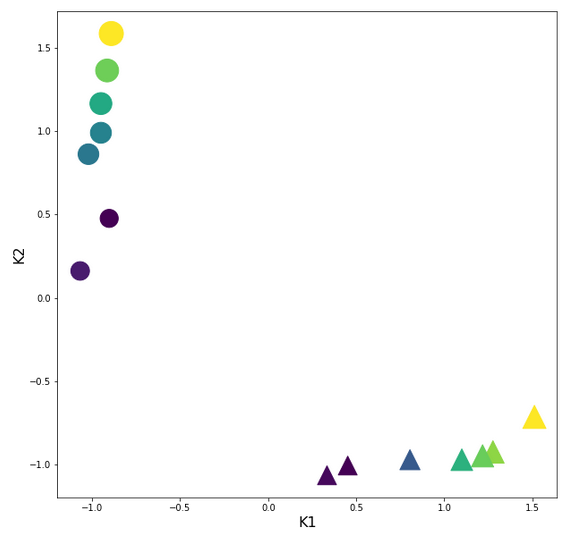You should recognize two things: The centralization of the features and the structural consistence of the clusters to the original distribution before scaling!

# The cost hyperplane over the {w1, w2}-space after the application of the StandardScaler to our input data

Let us apply the StandardScaler and look at the resulting cost hyperplane. When we set the parameters for a mesh display to

wm1 = np.arange(-5.0,5.0,0.002), wm2 = np.arange(-5.0,5.0,0.002)

we get the following results:

```C_min =  0.0006239618496774544
Coordinates:  [[2695 2259]]
Weight values at cost minimum: -0.4820000000004976 0.3899999999994064
```

Plots for total costs over the {w1, w2}-space from different angles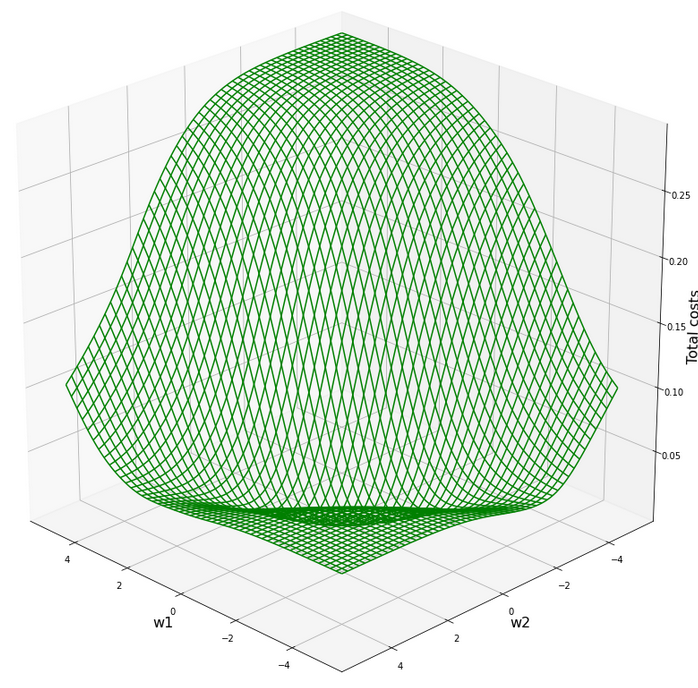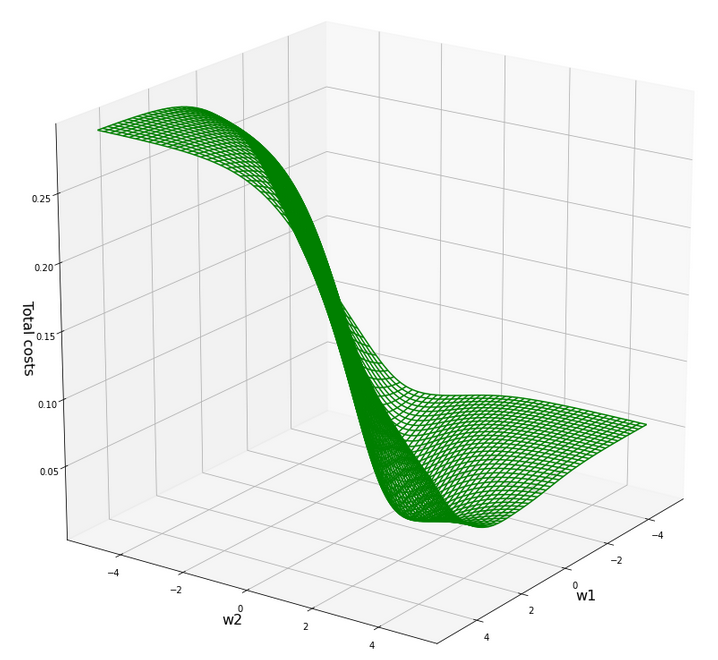Plot for individual costs (i=0, i=5) over the {w1, w2}-space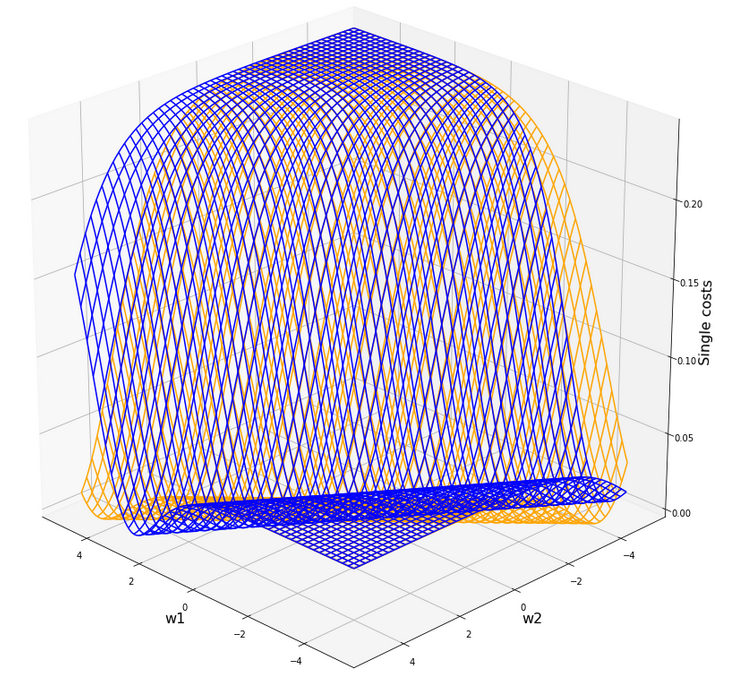The index "i" refers to our sample-array (see the last article).

# Gradient descent after scaling with the "StandardScaler"

Ok, let us now try gradient descent again. We set the following parameters:

w1_start = -0.20, w2_start = 0.25 eta = 0.1, decrease_rate = 0.000001, num_steps = 2000

Results:

```Stoachastic Descent
Kt1       Kt2     K1     K2  Tgt       Res       Err
0   1.276259 -0.924692  200.0   14.0  0.3  0.273761  0.087463
1  -1.067616  0.160925    1.0  107.0  0.7  0.640346  0.085220
2   0.805129 -0.971385  160.0   10.0  0.3  0.317122  0.057074
3  -0.949833  1.164828   11.0  193.0  0.7  0.713461  0.019230
4   1.511825 -0.714572  220.0   32.0  0.3  0.267573  0.108090
5  -0.949833  0.989729   11.0  178.0  0.7  0.699278  0.001031
6   0.333998 -1.064771  120.0    2.0  0.3  0.359699  0.198995
7  -0.914498  1.363274   14.0  210.0  0.7  0.725667  0.036666
8   1.217368 -0.948038  195.0   12.0  0.3  0.277602  0.074660
9  -0.902720  0.476104   15.0  134.0  0.7  0.650349  0.070930
10  0.451781 -1.006405  130.0    7.0  0.3  0.351926  0.173086
11 -1.020503  0.861322    5.0  167.0  0.7  0.695876  0.005891
12  1.099585 -0.971385  185.0   10.0  0.3  0.287246  0.042514
13 -0.890942  1.585067   16.0  229.0  0.7  0.740396  0.057709

Batch Descent
Kt1       Kt2     K1     K2  Tgt       Res       Err
0   1.276259 -0.924692  200.0   14.0  0.3  0.273755  0.087482
1  -1.067616  0.160925    1.0  107.0  0.7  0.640352  0.085212
2   0.805129 -0.971385  160.0   10.0  0.3  0.317118  0.057061
3  -0.949833  1.164828   11.0  193.0  0.7  0.713465  0.019236
4   1.511825 -0.714572  220.0   32.0  0.3  0.267566  0.108113
5  -0.949833  0.989729   11.0  178.0  0.7  0.699283  0.001025
6   0.333998 -1.064771  120.0    2.0  0.3  0.359697  0.198990
7  -0.914498  1.363274   14.0  210.0  0.7  0.725670  0.036672
8   1.217368 -0.948038  195.0   12.0  0.3  0.277597  0.074678
9  -0.902720  0.476104   15.0  134.0  0.7  0.650354  0.070923
10  0.451781 -1.006405  130.0    7.0  0.3  0.351924  0.173080
11 -1.020503  0.861322    5.0  167.0  0.7  0.695881  0.005884
12  1.099585 -0.971385  185.0   10.0  0.3  0.287241  0.042531
13 -0.890942  1.585067   16.0  229.0  0.7  0.740400  0.057714

Total error stoch descent:  0.07275422919538276
Total error batch descent:  0.07275715820661666
```

The attentive reader has noticed that I extended my code to include the columns with the original (K1, K2)-values into the Pandas dataframe. The code of the new function "predict_batch()" is given below. Do not forget to change the function calls at the end of the gradient descent code, too.

Now we obviously can speak of a result! The calculated (w1, w2)-data are:

```Final (w1,w2)-values stoch : ( -0.4816 ,  0.3908 )
Final (w1,w2)-values batch : ( -0.4815 ,  0.3906 )
```

Yeah, this is pretty close to the values we got via the fine grained mesh analysis of the cost function before! And within the error range!

Changed code for two of our functions in the last article

```def predict_batch(num_samples, w1, w2, ay_k_1, ay_k_2, li_K1, li_K2, li_a_tgt):
shape_res = (num_samples, 7)
ResData = np.zeros(shape_res)
rg_idx = range(num_samples)
err = 0.0
for idx in rg_idx:
z_in  = w1 * ay_k_1[idx] + w2 * ay_k_2[idx]
a_out = expit(z_in)
a_tgt = li_a_tgt[idx]
err_idx = np.absolute(a_out - a_tgt) / a_tgt
err += err_idx
ResData[idx] = ay_k_1[idx]
ResData[idx] = ay_k_2[idx]
ResData[idx] = li_K1[idx]
ResData[idx] = li_K2[idx]
ResData[idx] = a_tgt
ResData[idx] = a_out
ResData[idx] = err_idx
err /= float(num_samples)
return err, ResData

def create_df(ResData):
''' ResData: Array with result values K1, K2, Tgt, A, rel.err
'''
cols=["Kt1", "Kt2", "K1", "K2", "Tgt", "Res", "Err"]
df = pd.DataFrame(ResData, columns=cols)
return df
```

# How does the epoch evolution after the application of the StandardScaler look like?

Let us plot the evolution for the stochastic gradient descent:

Cost and weight evolution during stochastic gradient descent

Ok, we see that despite convergence the difference in the costs for different samples cannot be eliminated. It should be clear to the reader, why, and that this was to be expected.

We also see that the total costs (calculated from the individual costs) seemingly converges much faster than the weight values! Our gradient descent path obviously follows a big slope into a rather flat valley first (see the plot of the total costs above). Afterwards there is a small gradient sideways and down into the real minimum - and it obviously takes some epochs to get there. We also understand that we have to keep up a significant "learning rate" to follow the gradient in the flat valley. In addition the following rule seems to be appropriate sometimes:

We must not only watch the cost evolution but also the weight evolution - to avoid stopping gradient descent too early!

We shall keep this in mind for experiments with real multi-layer "Artificial Neural Networks" later on!

And how does the gradient descent based on the full "batch" of 14 samples look like?

Cost and weight evolution during batch gradient descent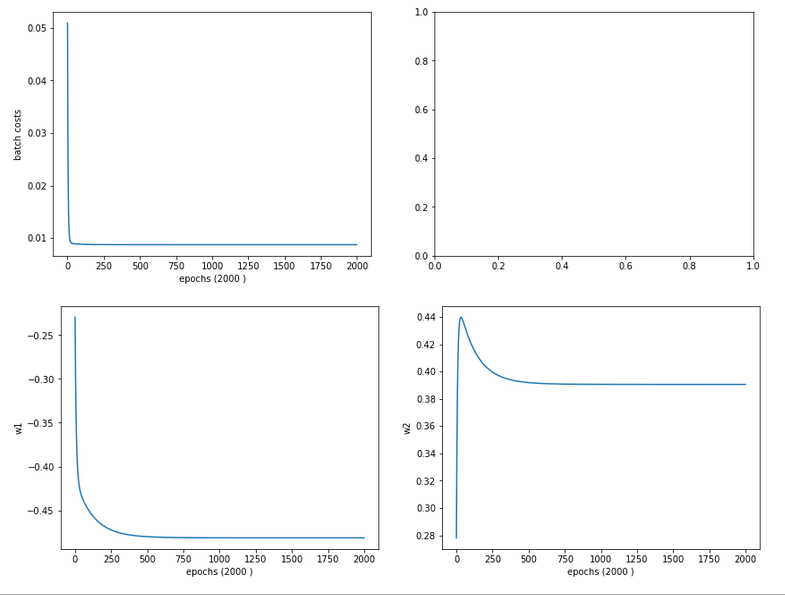A smooth beauty!

# Contour plot for separation curves in the {K1, K2}-plane

We add the following code to our Jupyter notebook:

```# ***********
# Contours
# ***********

from matplotlib import ticker, cm

# Take w1/w2-vals from above w1f, w2f
w1_len = len(li_w1_ba)
w2_len = len(li_w1_ba)
w1f = li_w1_ba[w1_len -1]
w2f = li_w2_ba[w2_len -1]

def A_mesh(w1,w2, Km1, Km2):
kshape = Km1.shape
A = np.zeros(kshape)

Km1V = Km1.reshape(kshape*kshape, )
Km2V = Km2.reshape(kshape*kshape, )
# print("km1V.shape = ", Km1V.shape, "\nkm1V.shape = ", Km2V.shape )

KmV = np.column_stack((Km1V, Km2V))

# scaling trafo
KmT = scaler.transform(KmV)

Km1T, Km2T = KmT.T
Km1TR = Km1T.reshape(kshape)
Km2TR = Km2T.reshape(kshape)
#print("km1TR.shape = ", Km1TR.shape, "\nkm2TR.shape = ", Km2TR.shape )

rg_idx = range(num_samples)
Z      = w1 * Km1TR + w2 * Km2TR
A = expit(Z)
return A

#Build K1/K2-mesh
minK1, maxK1 = li_K1.min()-20, li_K1.max()+20
minK2, maxK2 = li_K2.min()-20, li_K2.max()+20
resolution = 0.1
Km1, Km2 = np.meshgrid( np.arange(minK1, maxK1, resolution),
np.arange(minK2, maxK2, resolution))

A = A_mesh(w1f, w2f, Km1, Km2 )

fig_size = plt.rcParams["figure.figsize"]
fig_size = 14
fig_size = 11
fig, ax = plt.subplots()
cmap=cm.PuBu_r
cmap=cm.RdYlBu
#cs = plt.contourf(X, Y, Z1, levels=25, alpha=1.0, cmap=cm.PuBu_r)
cs = ax.contourf(Km1, Km2, A, levels=25, alpha=1.0, cmap=cmap)
cbar = fig.colorbar(cs)
N = 14
r0 = 0.6
x = li_K1
y = li_K2
area = 6*np.sqrt(x ** 2 + y ** 2)  # 0 to 10 point radii
c = np.sqrt(area)
r = np.sqrt(x ** 2 + y ** 2)
area1 = np.ma.masked_where(x < 100, area)
area2 = np.ma.masked_where(x >= 100, area)
ax.scatter(x, y, s=area1, marker='^', c=c)
ax.scatter(x, y, s=area2, marker='o', c=c)
# Show the boundary between the regions:
ax.set_xlabel("K1", fontsize=16)
ax.set_ylabel("K2", fontsize=16)

```

This code enables us to plot contours of predicted output values of our solitary neuron, i.e. A-values, on a mesh of the original {K1, K2}-plane. As we classified after a transformation of our input data, the following hint should be obvious:

Important hint: Of course you have to apply your scaling method to all the new input data created by the mesh-function! This is done in the above code in the "A_mesh()"-function with the following lines:

```    # scaling trafo
if (scale_method == 3):
KmT = scaler.fit_transform(KmV)
else:
KmT = scaler.transform(KmV)
```

We can directly apply the StandardScaler on our new data via its method transform(); the scaler will use the parameters it found during his first "scaler.fit_transform()"-operation on our input samples. However, we cannot do it this way when using the Normalizer for each individual new data sample via "scale_method =3". I shall come back to this point in a later article.

The careful reader also sees that our code will, for the time being, not work for scale_method=0, scale_method=2 and scale_method=5. Reason: I was too lazy to write a class or code suitable for these normalizing operations. I shall correct this when we need it.

But at least I added our input samples via scatter plotting to the final output. The result is: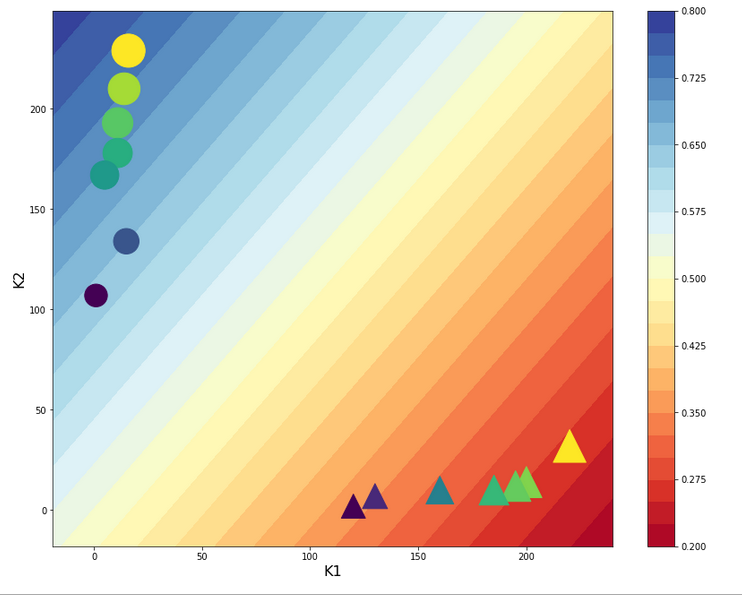The deviations from our target values is to be expected. With a given pair of (w1, w2)-values we cannot do much better with a single neuron and a linear weight impact on the input data.

But we see: If we set up a criterion like:

• A > 0.5 => sample belongs to the left cluster,
• A ≤ 0.5 => sample belongs to the right cluster

we would have a relatively good classificator available - based on one neuron only!

# Intermediate Conclusion

In this article I have shown that the "standardization" of input data, which are fed into a perceptron ahead of a gradient descent calculation, helps to circumvent problems with the saturation of the sigmoid function at the computing neuron following the input layer. We achieved this by applying the "StandardScaler" of Scikit-Learn. We got a smooth development of both the cost function and the weight parameters during gradient descent in the transformed data space.

We also learned another important thing:

An apparent convergence of the cost function in the vicinity of a minimum value does not always mean that we have reached the global minimum, yet. The evolution of the weight parameters may not yet have come to an end! Therefore, it is important to watch both the evolution of the costs AND the evolution of the weights during gradient descent. A too fast decline of the learning rate may not be good either under certain conditions.

In the next article

A single neuron perceptron with sigmoid activation function – III – two ways of applying Normalizer

we shall look at two other normalization methods for our simplistic scenario. One of them will give us an even better classificator.

Stay tuned and remain healthy ...

And Mr Trump:
One neuron can obviously learn something about the difference of big and small numbers. This leads me to two questions, which you as a "natural talent" on epidemics can certainly answer: How many neurons are necessary to understand something about an exponential epidemic development? And why did it take so much time to activate them?

# A single neuron perceptron with sigmoid activation function – I – failure of gradient descent due to saturation

Readers who follow my series on a Python program for a "Multilayer Perceptron" [MLP] have noticed that I emphasized the importance of a normalization of input data in my last article:

A simple Python program for an ANN to cover the MNIST dataset – XII – accuracy evolution, learning rate, normalization

Our MLP "learned" by searching for the global minimum of the loss function via the "gradient descent" method. Normalization seemed to improve a smooth path to convergence whilst our algorithm moved into the direction of a global minimum on the surface of the loss or cost functions hyperplane over the weight parameter space. At least in our case where we used the sigmoid function as the activation function of the artificial neurons. I indicated that the reason for our observations had to do with properties of this function - especially for neurons in the first hidden layer of an MLP.

In case of the 4-layer MLP, which I used on the MNIST dataset, I applied a special form of normalization namely "standardization". I did this by using the StandarScaler of SciKit-Learn. See the following link for a description: Sklearn preprocessing StandardScaler

We saw the smoothing and helpful impact of normalization on the general convergence of gradient descent by the help of a few numerical experiments. The interaction of the normalization of 784 features with mini-batches and with a complicated 4 layer-MLP structure, which requires the determination of several hundreds of thousands weight values is however difficult to grasp or analyze. One understands that there is a basic relation to the properties of the activation function, but the sheer number of the dimensions of the feature and weight spaces and statistics make a thorough understanding difficult.

Since then I have thought a bit about how to set up a really small and comprehensible experiment which makes the positive impact of normalization visible in a direct and visual form. I have come up with the following most simple scenario, namely the training of a simple perceptron with only one computing neuron and two additional "stupid" neurons in an input layer which just feed input data to our computing neuron: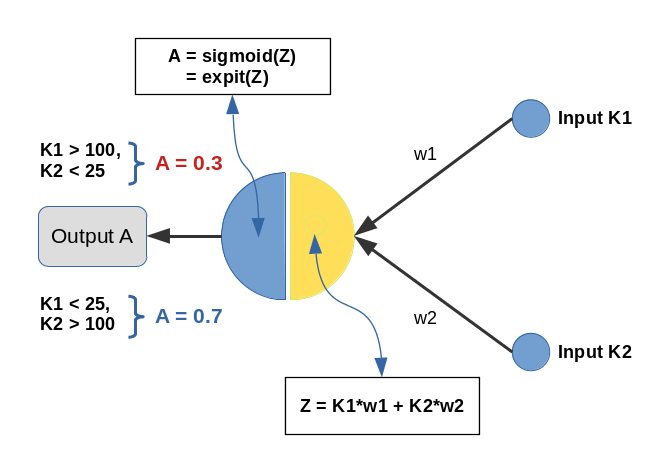Input values K1 and K2 are multiplied by weights w1 and w2 and added by the central solitary "neuron". We use the sigmoid function as the "activation function" of this neuron - well anticipating that the properties of this function may lead to trouble.

The perceptron has only one task: Discriminate between two different types of input data by assigning them two distinct output values.

• For K1 > 100 and K2 < 25 we want an output of A=0.3.
• For K1 &l; 25 and K2 > 100 we, instead, want an output of A=0.7

We shall feed the perceptron only 14 different pairs of input values K1[i], K2[i] (i =0,1,..13), e.g. in form of lists:

```li_K1 = [200.0,   1.0, 160.0,  11.0, 220.0,  11.0, 120.0,  22.0, 195.0,  15.0, 130.0,   5.0, 185.0,  16.0]
li_K2 = [ 14.0, 107.0,  10.0, 193.0,  32.0, 178.0,   2.0, 210.0,  12.0, 134.0,  15.0, 167.0,  10.0, 229.0]
```

(The careful reader detects one dirty example li_K2 = 32 (> 25), in contrast to our setting. Just to see how much of an impact such a deviation has ...)

We call each pair (K1, K2)=(li_K1[i], li_K2[i]) for a give "i" a "sample". Each sample contains values for two "features": K1 and K2. So, our solitary computing neuron has to solve a typical classification problem - it shall distinguish between two groups of input samples. In a way it must learn the difference between small and big numbers for 2 situations appearing at its input channels.

Off topic: This morning I listened to a series of comments of Mr. Trump during the last weeks on the development of the Corona virus crisis in the USA. Afterwards, I decided to dedicate this mini-series of articles on a perceptron to him - a person who claims to "maybe" be "natural talent" on complicated things as epidemic developments. Enjoy (?) his own words via an audio compilation in the following news article:
https://www.faz.net/aktuell/politik/trumps-praesidentschaft/usa-zehn-wochen-corona-in-den-worten-von-trump-16708603.html

# Two well separable clusters

In the 2-dim feature space {K1, K2} we have just two clusters of input data: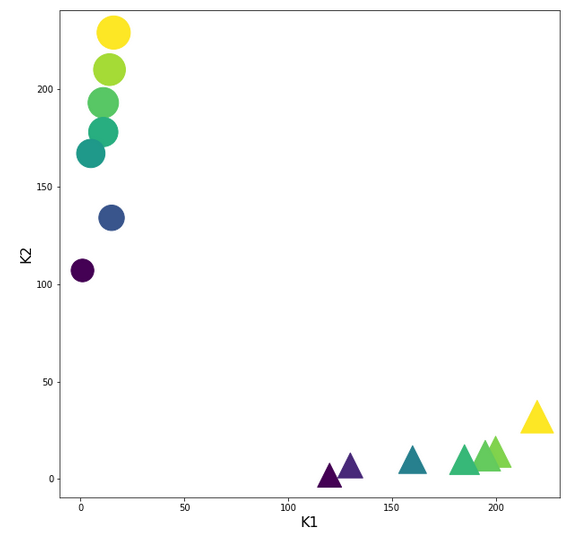Each cluster has a long diameter along one of the feature axes - but overall the clusters are well separable. A rather good separation surface would e.g. be a diagonal line.

For a given input sample with K-values K1 and K2 we define the output of our computing neuron to be

A(w1, w2) = expit( w1*K1 + w2*K2 ) ,

where expit() symbolizes the sigmoid function. See the next section for more details.

Corresponding target-values for the output A are (with at1 = 0.3 and at2 = 0.7):

```li_a_tgt = [at1,  at2,  at1,  at2,  at1,  at2,  at1,  at2,  at1,  at2,  at1,  at2,   at1,  at2]
```

With the help of these target values our poor neuron shall learn from the 14 input samples to which cluster a given future sample probably belongs to. We shall use the "gradient descent" method to train the neuron for this classification task. o solve the task our neuron must find a reasonable separation surface - a line - in the {K1,K2}-plane; but it got the additional task to associate two distinct output values "A" with the two clusters:

A=0.7 for data points with a large K1-value and A=0.3 for data points with a small K1-value.

So, the separation surface has to fulfill some side conditions.

Readers with a background in Machine Learning and MLPs will now ask: Why did you pick the special values 0.3 and 0.7? A good question - I will come back to it during our experiments. Another even more critical question could be: What about a bias neuron in the input layer? Don't we need it? Again, a very good question! A bias neuron allows for a shift of a separation surface in the feature space. But due to the almost symmetrical nature of our input data (see the positions and orientations of the clusters!) and the target values the impact of a bias neuron on the end result would probably only be small - but we shall come back to the topic of a bias neuron in a later article. But you are free to extend the codes given below to account for a bias neuron in the input layer. You will notice a significant impact if you change either the relative symmetry of the input or of the output data. But lets keep things simple for the next hours ...

# The sigmoid function and its saturation for big arguments

You can import the sigmoid function under the name "expit" from the "scipy" library into your Python code. The sigmoid function is a smooth one - but it quickly saturates for big negative or positive values: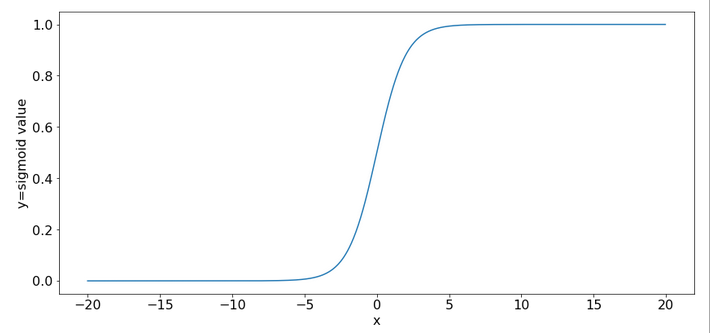So, output values get almost indistinguishable if the absolute values of the arguments are bigger than 15.

# What is interesting about our input data? What is the relation to MNIST data?

The special situation about the features in our example is the following: For one and the same feature we have a big number and a small number to work with - depending on the sample. Which feature value - K1 or K2 - is big depends on the sample.

This is something that also happens with the input "features" (i.e. pixels) coming from a MNIST-image:
For a certain feature (= a pixel at a defined position in the 28x28 picture) in a given sample (= a distinct image) we may get a big number as 255. For another feature (= pixel) the situation may be totally different and we may find a value of 0. In another sample (= picture) we may get the reverse situation for the same two pixels.

What happens in such a situation at a specific neuron in the first hidden neuron layer of a MLP when we start gradient descent with a statistical distribution of weight values? If we are unlucky then the initial statistical weight constellation for a sample may lead to a big number of the total input to our selected hidden neuron with the effect of a very small gradient at this node - due to saturation of the sigmoid function.

To give you a feeling: Assume that you have statistical weights in the range of [-0.025, 0.025]. Assume further that only 4 pixels of a MNIST picture with a value of 200 contribute with a local maximum weight of 0.25; then we get a a minimum input at our node of 4*0.25*200 = 20. The gradient of expit(20) has a value of 2e-9. Even if we multiply by the required factor of 200 for a weight correction at one of the contributing input nodes we would would arrive at 4e-7. Quite hopeless. Of course, the situation is not that bad for all weights and image-samples, but you get an idea about the basic problem ....

Our simple scenario breaks the MNIST situation down to just two features and just one working neuron - and therefore makes the correction situation for gradient descent really extreme - but interesting, too. And we can analyze the situation far better than for a MLP because we deal with an only 2-dimensional feature-space {K1, K2} and a 2-dimensional weight-space {w1, w2}.

# A simple quadratic cost function for our neuron

For given values w1 and w2, i.e. a tuple (w1, w2), we define a quadratic cost or loss function C_sgl for one single input sample (K1, K2) as follows:

C_sgl = 0.5 * ( li_a_tgt(i) - expit(z_i) )**2, with z_i = li_K[i]*w1 + li_K2[i]*w2

The total cost-function for the batch of all 14 samples is just the sum of all these terms for the individual samples.

# Existence of a solution for our posed problem?

From all we theoretically know about the properties of a simple perceptron it should be able to find a reasonable solution! But, how do we know that a reasonable solution for a (w1, w2)-pair does exist at all? One line of reasoning goes like follows:

For two samples - each a member of either cluster - you can plot the hyperplanes of the related outputs "A(K1, K2) = expit(w1*K1+w2*K2)" over the (w1, w2)-space. These hyperplanes are almost orthogonal to each other. If you project the curves of a cut with the A=0.3-planes and the A=0.7-planes down to the (w1, w2)-plane at the center you get straight lines - almost orthogonally oriented against each other. So, such 2 specific lines cut each other in a well defined point - somewhere off the center. As the expit()-function is a relatively steep one for our big input values the crossing point is relatively close to the center. If we choose other samples we would get slightly different lines and different crossing points of the projected lines - but not too far from each other.

The next plot shows the expit()-functions close to the center of the (w1, w2)-plane for two specific samples of either cluster. We have in addition displayed the surfaces for A=0.7 and A=0.3.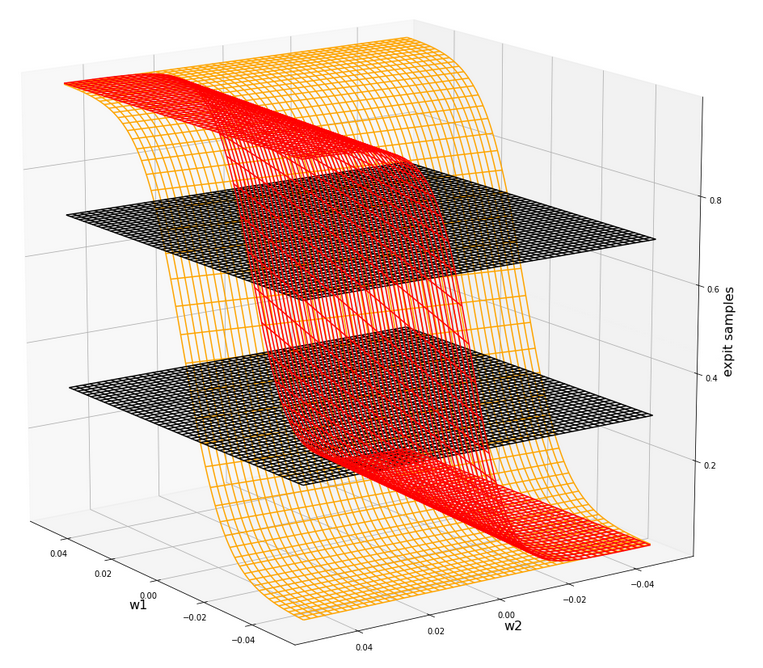The following plot shows the projections of the cuts of the surfaces for 7 samples of each cluster with the A=0.3-plane and the A=0.7, respectively.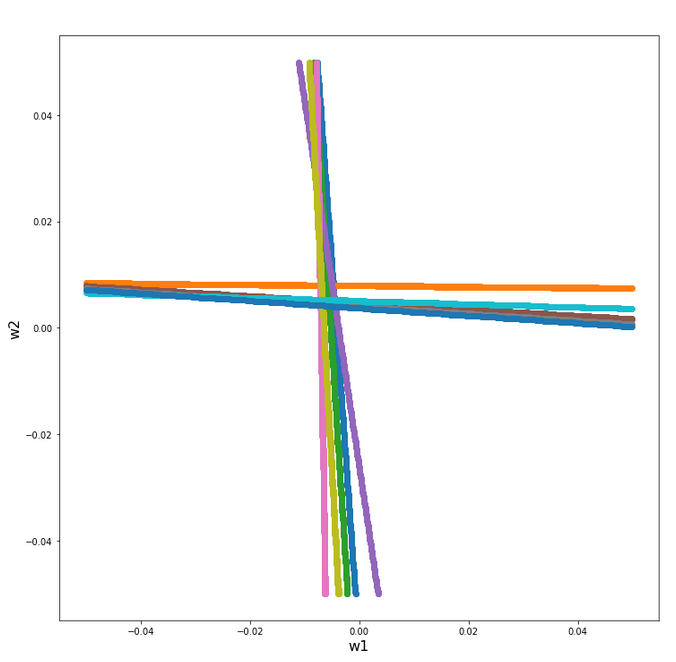The area of crossings is not too big on the chosen scale of w1, w2. Looking at the graphics we would expect an optimal point around (w1=-0.005, w2=+0.005) - for the original, unnormalized input data.

By the way: There is also a solution for at1=0.3 and at2=0.3, but a strange one. Such a setup would not allow for discrimination. We expect a rather strange behavior then. A first guess could be: The resulting separation curve in the (K1, K2)-plane would move out of the area between the two clusters.

# Code for a mesh based display of the costs over the weight-parameter space

Below you find the code suited for a Jupyter cell to get a mesh display of the cost values

```import numpy as np
import numpy as np
import random
import math
import sys

from sklearn.preprocessing import StandardScaler
from sklearn.preprocessing import Normalizer
from sklearn.preprocessing import MinMaxScaler
from scipy.special import expit

import matplotlib as mpl
from matplotlib import pyplot as plt
from matplotlib.colors import ListedColormap
import matplotlib.patches as mpat
from mpl_toolkits import mplot3d
from mpl_toolkits.mplot3d import Axes3D

# total cost functions for overview
# *********************************
def costs_mesh(num_samples, W1, W2, li_K1, li_K2, li_a_tgt):
zshape = W1.shape
li_Z_sgl = []
li_C_sgl = []
C = np.zeros(zshape)

rg_idx = range(num_samples)
for idx in rg_idx:
Z_idx      = W1 * li_K1[idx] + W2 * li_K2[idx]
A_tgt_idx  = li_a_tgt[idx] * np.ones(zshape)
C_idx = 0.5 * ( A_tgt_idx - expit(Z_idx) )**2
li_C_sgl.append( C_idx )
C += C_idx

C /= np.float(num_samples)
return C, li_C_sgl

# ******************
# Simple Perceptron
#*******************

# input at 2 nodes => 2 features K1 and K2 => there will be just one output neuron
li_K1 = [200.0,   1.0, 160.0,  11.0, 220.0,  11.0, 120.0,  14.0, 195.0,  15.0, 130.0,   5.0, 185.0,  16.0  ]
li_K2 = [ 14.0, 107.0,  10.0, 193.0,  32.0, 178.0,   2.0, 210.0,  12.0, 134.0,  7.0, 167.0,  10.0, 229.0 ]

# target values
at1 = 0.3; at2 = 0.7
li_a_tgt  = [at1,  at2,  at1,  at2,   at1,   at2,  at1,   at2,  at1,  at2,  at1,  at2,   at1,  at2 ]

# Change to np floats
li_K1 = np.array(li_K1)
li_K2 = np.array(li_K2)
li_a_tgt = np.array(li_a_tgt)

num_samples = len(li_K1)

# Get overview over costs on mesh
# *******************************
# Mesh of weight values
wm1 = np.arange(-0.2,0.4,0.002)
wm2 = np.arange(-0.2,0.2,0.002)
W1, W2 = np.meshgrid(wm1, wm2)
# costs
C, li_C_sgl  = costs_mesh(num_samples=num_samples, W1=W1, W2=W2, \
li_K1=li_K1, li_K2=li_K2, li_a_tgt = li_a_tgt)

# Mesh-Plots
# ********
fig_size = plt.rcParams["figure.figsize"]
print(fig_size)
fig_size = 19
fig_size = 19

fig1 = plt.figure(1)
fig2 = plt.figure(2)

ax1 = fig1.gca(projection='3d')
ax1.get_proj = lambda: np.dot(Axes3D.get_proj(ax1), np.diag([1.0, 1.0, 1, 1]))
ax1.view_init(15,148)
ax1.set_xlabel('w1', fontsize=16)
ax1.set_ylabel('w2', fontsize=16)
ax1.set_zlabel('single costs', fontsize=16)

#ax1.plot_wireframe(W1, W2, li_C_sgl, colors=('blue'))
#ax1.plot_wireframe(W1, W2, li_C_sgl, colors=('orange'))
ax1.plot_wireframe(W1, W2, li_C_sgl, colors=('orange'))
ax1.plot_wireframe(W1, W2, li_C_sgl, colors=('green'))
#ax1.plot_wireframe(W1, W2, li_C_sgl, colors=('orange'))
#ax1.plot_wireframe(W1, W2, li_C_sgl, colors=('magenta'))

ax2 = fig2.gca(projection='3d')
ax2.get_proj = lambda: np.dot(Axes3D.get_proj(ax2), np.diag([1.0, 1.0, 1, 1]))
ax2.view_init(15,140)
ax2.set_xlabel('w1', fontsize=16)
ax2.set_ylabel('w2', fontsize=16)
ax2.set_zlabel('Total costs', fontsize=16)
ax2.plot_wireframe(W1, W2, 1.2*C, colors=('green'))

```

# The cost landscape for individual samples without normalization

Gradient descent tries to find a minimum of a cost function by varying the weight values systematically in the cost gradient's direction. To get an overview about the cost hyperplane over the 2-dim (w1, w2)-space we make some plots. Let us first plot 2 the individual costs for the input samples i=0 and i=5.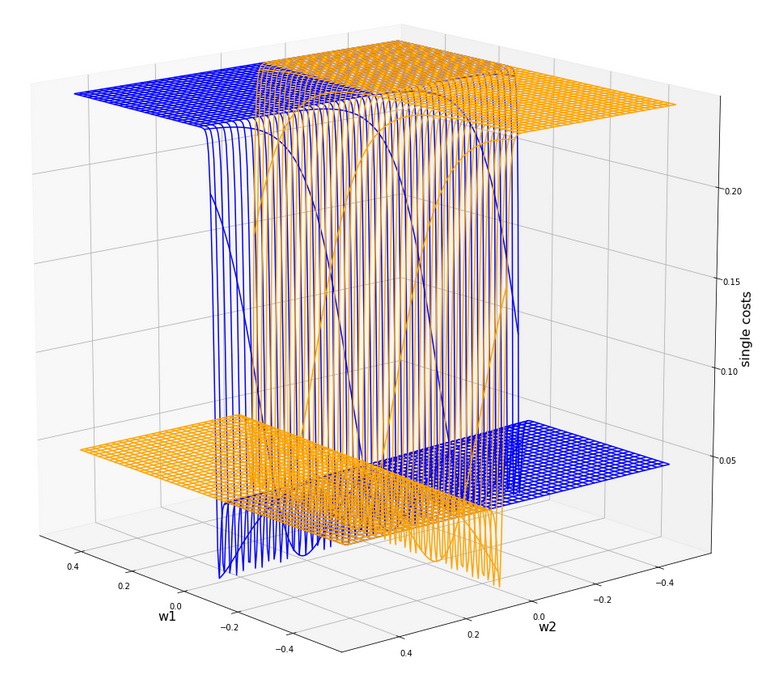Actually the cost functions for the different samples do show some systematic, though small differences. Try it out yourself ... Here is the plot for samples 1,5,9 (counted from 0!).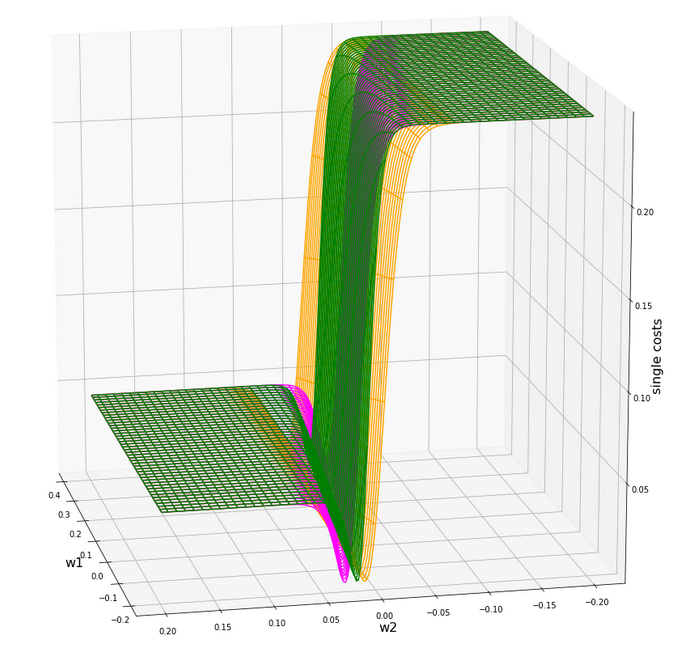You see different orientation angles in the (w1, w2)-plane?

# Total cost landscape without normalization

Now let us look at the total costs; to arrive at a comparable level with the other plots I divided the sum by 14: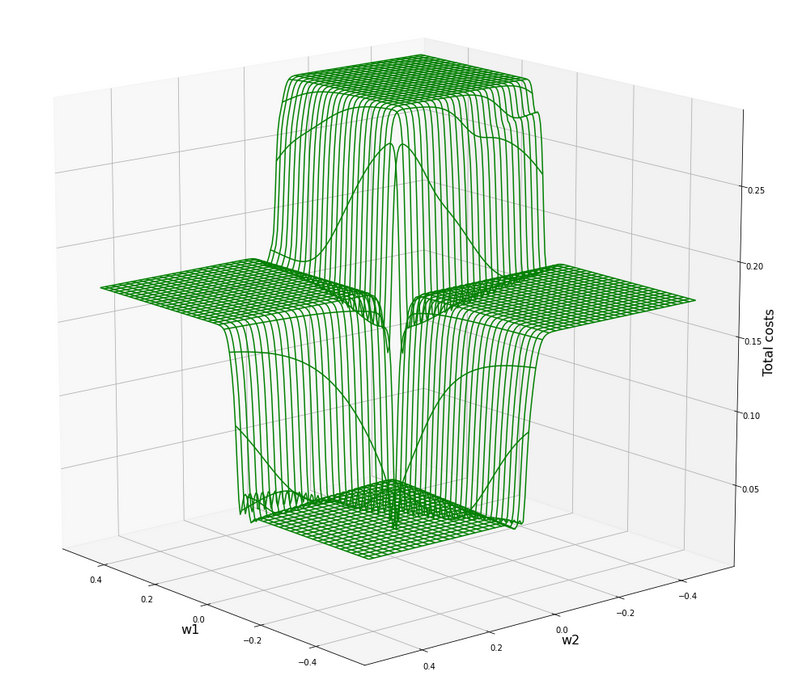All of the above cost plots look like big trouble for both the "stochastic gradient descent" and the "batch gradient descent" methods for "Machine Learning" [ML]:

We clearly see the effect of the sigmoid saturation. We get almost flat areas beyond certain relatively small w1- and w2-values (|w1| > 0.02, |w2| > 0.02). The gradients in this areas will be very, very close to zero. So, if we have a starting point as e.g. (w1=0.3, w2=0.2) our gradient descent would get stuck. Due to the big input values of at least one feature.

In the center of the {w1, w2}-plane, however, we detect a steep slope to a global minimum.

But how to get there? Let us say, we start with w1=0.04, w2=0.04. The learning-rate "η" is used to correct the weight values by

In the beginning you would need a big "η" to get closer to the center due to small gradient values. However, if you choose it too big you may pass the tiny area of the minimum and just hop to an area of a different cost level with again a gradient close to zero. But you cannot decrease the learning rate fast as a remedy, either, to avoid getting stuck again.

# A view at the center of the loss function hyperplane

Let us take a closer look at the center of our disastrous total cost function. We can get there by reducing our mesh to a region defined by "-0.05 < w1, w2 < 0.05". We get :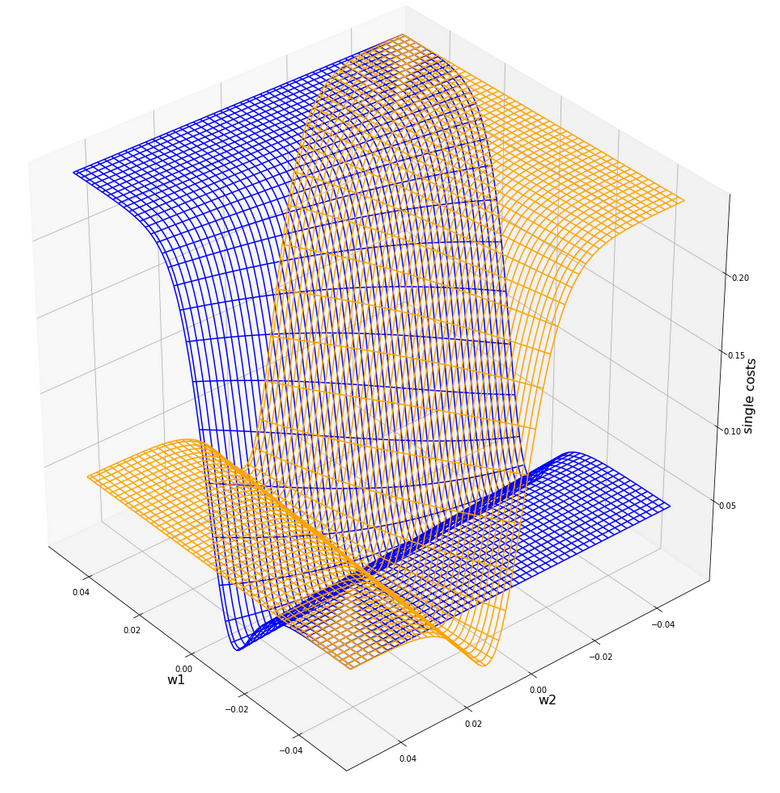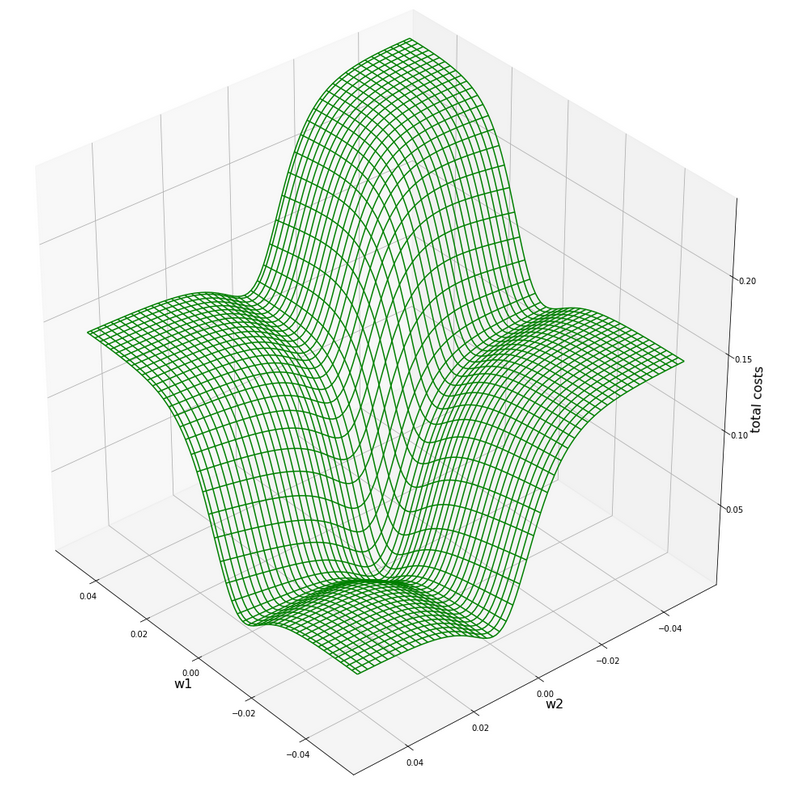This looks actually much better - on such a surface we could probably work with gradient descent. There is a clear minimum visible - and on this scale of the (w1, w2)-values we also recognize reasonably paths into this minimum. An analysis of the meshdata to get values for the minimum is possible by the following statements:

```print("min =", C.min())
pt_min = np.argwhere(C==C.min())
w1=W1[pt_min][pt_min]
w2=W2[pt_min][pt_min]
print("w1 = ", w1)
print("w2 = ", w2)
```

The result is:

min = 0.0006446277000906343
w1 = -0.004999999999999963
w2 = 0.005000000000000046

But to approach this minimum by a smooth gradient descent we would have had to know in advance at what tiny values of (w1, w2) to start with gradient descent - and choose our η suitably small in addition. This is easy in our most simplistic one neuron case, but you almost never can fulfill the first condition when dealing with real artificial neural networks for complex scenarios.

And a naive gradient descent with a standard choice of a (w1, w2)-starting point would have lead us to nowhere in our one-neuron case - as we shall see in a minute ..

Let us keep one question in mind for a while: Is there a chance that we could get the hyperplane surface to look similar to the one at the center - but for much bigger weight values?

# Some Python code for gradient descent for our one neuron scenario

Here are some useful functions, which we shall use later on to perform a gradient descent:

```
# ****************************************
# Functions for stochastic GRADIENT DESCENT
# *****************************************
import random
import pandas as pd

# derivative of expit
def d_expit(z):
exz = expit(z)
dex = exz * (1.0 - exz)
return dex

# single costs for stochastic descent
# ************************************
def dcost_sgl(w1, w2, idx, li_K1, li_K2, li_a_tgt):
z_in  = w1 * li_K1[idx] + w2 * li_K2[idx]
a_tgt = li_a_tgt[idx]
c = 0.5 * ( a_tgt - expit(z_in))**2
return c

# *********
def grad_sgl(w1, w2, idx, li_K1, li_K2, li_a_tgt):
z_in  = w1 * li_K1[idx] + w2 * li_K2[idx]
a_tgt = li_a_tgt[idx]
gradw1 = 0.5 * 2.0 * (a_tgt - expit(z_in)) * (-d_expit(z_in)) * li_K1[idx]
gradw2 = 0.5 * 2.0 * (a_tgt - expit(z_in)) * (-d_expit(z_in)) * li_K2[idx]

def grad_tot(num_samples, w1, w2, li_K1, li_K2, li_a_tgt):
rg_idx = range(num_samples)
for idx in rg_idx:
z_in  = w1 * li_K1[idx] + w2 * li_K2[idx]
a_tgt = li_a_tgt[idx]
gradw1_idx = 0.5 * 2.0 * (a_tgt - expit(z_in)) * (-d_expit(z_in)) * li_K1[idx]
gradw2_idx = 0.5 * 2.0 * (a_tgt - expit(z_in)) * (-d_expit(z_in)) * li_K2[idx]

# total costs at given point
# ************************************
def dcost_tot(num_samples, w1, w2,li_K1, li_K2, li_a_tgt):
c_tot  = 0
rg_idx = range(num_samples)
for idx in rg_idx:
#z_in  = w1 * li_K1[idx] + w2 * li_K2[idx]
a_tgt = li_a_tgt[idx]
c_idx = dcost_sgl(w1, w2, idx, li_K1, li_K2, li_a_tgt)
c_tot += c_idx
ctot = 1.0/num_samples * c_tot
return c_tot

# Prediction function
# ********************
def predict_batch(num_samples, w1, w2,ay_k_1, ay_k_2, li_a_tgt):
shape_res = (num_samples, 5)
ResData = np.zeros(shape_res)
rg_idx = range(num_samples)
err = 0.0
for idx in rg_idx:
z_in  = w1 * ay_k_1[idx] + w2 * ay_k_2[idx]
a_out = expit(z_in)
a_tgt = li_a_tgt[idx]
err_idx = np.absolute(a_out - a_tgt) / a_tgt
err += err_idx
ResData[idx] = ay_k_1[idx]
ResData[idx] = ay_k_2[idx]
ResData[idx] = a_tgt
ResData[idx] = a_out
ResData[idx] = err_idx
err /= float(num_samples)
return err, ResData

def predict_sgl(k1, k2, w1, w2):
z_in  = w1 * k1 + w2 * k2
a_out = expit(z_in)
return a_out

def create_df(ResData):
''' ResData: Array with result values K1, K2, Tgt, A, rel.err
'''
cols=["K1", "K2", "Tgt", "Res", "Err"]
df = pd.DataFrame(ResData, columns=cols)
return df

```

With these functions a quick and dirty "gradient descent" can be achieved by the following code:

```
# **********************************
# Quick and dirty Gradient Descent
# **********************************
b_scale_2 = False
if b_scale_2:
ay_k_1 = ay_K1
ay_k_2 = ay_K2
else:
ay_k_1 = li_K1
ay_k_2 = li_K2

li_w1_st = []
li_w2_st = []
li_c_sgl_st = []
li_c_tot_st = []

li_w1_ba = []
li_w2_ba = []
li_c_sgl_ba = []
li_c_tot_ba = []

idxc = 2

# Starting point
#***************
w1_start = -0.04
w2_start = -0.0455
#w1_start = 0.5
#w2_start = -0.5

# learn rate
# **********
eta = 0.0001
decrease_rate = 0.000000001
num_steps = 2500

# norm = 1
#eta = 0.75
#decrease_rate = 0.000000001
#num_steps = 100

# *********************
rg_j = range(num_steps)
rg_i = range(num_samples)
w1d_st = w1_start
w2d_st = w2_start
w1d_ba = w1_start
w2d_ba = w2_start

for j in rg_j:
eta = eta / (1.0 + float(j) * decrease_rate)
# loop over samples and individ. corrs
ns = num_samples
rg = range(ns)
rg_idx = random.sample(rg, num_samples)
#print("\n")
for idx in rg_idx:
#print("idx = ", idx)
li_w1_st.append(w1d_st)
li_w2_st.append(w2d_st)

# costs for special sample
cd_sgl_st = dcost_sgl(w1d_st, w2d_st, idx, ay_k_1, ay_k_2, li_a_tgt)
li_c_sgl_st.append(cd_sgl_st)

# total costs for special sample
cd_tot_st = dcost_tot(num_samples, w1d_st, w2d_st, ay_k_1, ay_k_2, li_a_tgt)
li_c_tot_st.append(cd_tot_st)
#print("j:", j, " li_c_tot[j] = ", li_c_tot[j] )

# work with total costs and total gradient
li_w1_ba.append(w1d_ba)
li_w2_ba.append(w2d_ba)
co_ba = dcost_tot(num_samples, w1d_ba, w2d_ba, ay_k_1, ay_k_2, li_a_tgt)
li_c_tot_ba.append(co_ba)

# Printed Output
# ***************
num_end = len(li_w1_st)
err_sgl, ResData_sgl = predict_batch(num_samples, li_w1_st[num_end-1], li_w2_st[num_end-1], ay_k_1, ay_k_2, li_a_tgt)
err_ba,  ResData_ba = predict_batch(num_samples, li_w1_ba[num_steps-1], li_w2_ba[num_steps-1], ay_k_1, ay_k_2, li_a_tgt)
df_sgl = create_df(ResData_sgl)
df_ba  = create_df(ResData_ba)
print("\n", df_sgl)
print("\n", df_ba)
print("\nTotal error stoch descent: ", err_sgl )
print("Total error batch descent: ", err_ba )

# Styled Pandas Output
# *******************
df_ba

```

Those readers who followed my series on a Multilayer Perceptron should have no difficulties to understand the code: I used two methods in parallel - one for a "stochastic descent" and one for a "batch descent":

• During "stochastic descent" we correct the weights by a stepwise application of the cost-gradients of single samples. (We shuffle the order of the samples statistically during epochs to avoid cyclic effects.) This is done for all samples during an epoch.
• During batch gradient we apply the gradient of the total costs of all samples once during each epoch.

And here is also some code to perform some plotting after training runs:

```
# Plots for Single neuron Gradient Descent
# ****************************************
#sizing
fig_size = plt.rcParams["figure.figsize"]
fig_size = 14
fig_size = 5

fig1 = plt.figure(1)
fig2 = plt.figure(2)
fig3 = plt.figure(3)
fig4 = plt.figure(4)

ax1_1.plot(range(len(li_c_sgl_st)), li_c_sgl_st)
#ax1_1.set_xlim (0, num_tot+5)
#ax1_1.set_ylim (0, 0.4)
ax1_1.set_xlabel("epochs * batches (" + str(num_steps) + " * " + str(num_samples) + " )")
ax1_1.set_ylabel("sgl costs")

ax1_2.plot(range(len(li_c_tot_st)), li_c_tot_st)
#ax1_2.set_xlim (0, num_tot+5)
#ax1_2.set_ylim (0, y_max_err)
ax1_2.set_xlabel("epochs * batches (" + str(num_steps) + " * " + str(num_samples) + " )")
ax1_2.set_ylabel("total costs ")

ax2_1.plot(range(len(li_w1_st)), li_w1_st)
#ax1_1.set_xlim (0, num_tot+5)
#ax1_1.set_ylim (0, y_max_costs)
ax2_1.set_xlabel("epochs * batches (" + str(num_steps) + " * " + str(num_samples) + " )")
ax2_1.set_ylabel("w1")

ax2_2.plot(range(len(li_w2_st)), li_w2_st)
#ax1_2.set_xlim (0, num_to_t+5)
#ax1_2.set_ylim (0, y_max_err)
ax2_2.set_xlabel("epochs * batches (" + str(num_steps) + " * " + str(num_samples) + " )")
ax2_2.set_ylabel("w2")

ax3_1.plot(range(len(li_c_tot_ba)), li_c_tot_ba)
#ax3_1.set_xlim (0, num_tot+5)
#ax3_1.set_ylim (0, 0.4)
ax3_1.set_xlabel("epochs (" + str(num_steps) + " )")
ax3_1.set_ylabel("batch costs")

ax4_1.plot(range(len(li_w1_ba)), li_w1_ba)
#ax4_1.set_xlim (0, num_tot+5)
#ax4_1.set_ylim (0, y_max_costs)
ax4_1.set_xlabel("epochs (" + str(num_steps) + " )")
ax4_1.set_ylabel("w1")

ax4_2.plot(range(len(li_w2_ba)), li_w2_ba)
#ax4_2.set_xlim (0, num_to_t+5)
#ax4_2.set_ylim (0, y_max_err)
ax4_2.set_xlabel("epochs (" + str(num_steps) + " )")
ax4_2.set_ylabel("w2")

```

You can put these codes into suitable cells of a Jupyter environment and start doing experiments on your PC.

# Frustration WITHOUT data normalization ...

Let us get the worst behind us:
Let us use un-normalized input data, set a standard starting point for the weights and try a gradient descent with 2500 epochs.
Well, what are standard initial weight values? We can follow LeCun's advice on bigger networks: a uniform distribution between - sqrt(1/2) and +srt(1/2) = 0.7 should be helpful. Well, we take such values. The parameters of our trial run are:

w1_start = -0.1, w2_start = 0.1, eta = 0.01, decrease_rate = 0.000000001, num_steps = 12500

You, unfortunately, get nowhere:

```        K1     K2  Tgt           Res       Err
0   200.0   14.0  0.3  3.124346e-15  1.000000
1     1.0  107.0  0.7  9.999996e-01  0.428571
2   160.0   10.0  0.3  2.104822e-12  1.000000
3    11.0  193.0  0.7  1.000000e+00  0.428571
4   220.0   32.0  0.3  1.117954e-15  1.000000
5    11.0  178.0  0.7  1.000000e+00  0.428571
6   120.0    2.0  0.3  8.122661e-10  1.000000
7    14.0  210.0  0.7  1.000000e+00  0.428571
8   195.0   12.0  0.3  5.722374e-15  1.000000
9    15.0  134.0  0.7  9.999999e-01  0.428571
10  130.0    7.0  0.3  2.783284e-10  1.000000
11    5.0  167.0  0.7  1.000000e+00  0.428571
12  185.0   10.0  0.3  2.536279e-14  1.000000
13   16.0  229.0  0.7  1.000000e+00  0.428571

K1     K2  Tgt           Res       Err
0   200.0   14.0  0.3  7.567897e-24  1.000000
1     1.0  107.0  0.7  1.000000e+00  0.428571
2   160.0   10.0  0.3  1.485593e-19  1.000000
3    11.0  193.0  0.7  1.000000e+00  0.428571
4   220.0   32.0  0.3  1.411189e-21  1.000000
5    11.0  178.0  0.7  1.000000e+00  0.428571
6   120.0    2.0  0.3  2.293804e-16  1.000000
7    14.0  210.0  0.7  1.000000e+00  0.428571
8   195.0   12.0  0.3  1.003437e-23  1.000000
9    15.0  134.0  0.7  1.000000e+00  0.428571
10  130.0    7.0  0.3  2.463730e-16  1.000000
11    5.0  167.0  0.7  1.000000e+00  0.428571
12  185.0   10.0  0.3  6.290055e-23  1.000000
13   16.0  229.0  0.7  1.000000e+00  0.428571

Total error stoch descent:  0.7142856616691734
Total error batch descent:  0.7142857142857143
```

A parameter setting like

w1_start = -0.1, w2_start = 0.1, eta = 0.0001, decrease_rate = 0.000000001, num_steps = 25000

does not bring us any further:

```        K1     K2  Tgt           Res       Err
0   200.0   14.0  0.3  9.837323e-09  1.000000
1     1.0  107.0  0.7  9.999663e-01  0.428523
2   160.0   10.0  0.3  3.496673e-07  0.999999
3    11.0  193.0  0.7  1.000000e+00  0.428571
4   220.0   32.0  0.3  7.812207e-09  1.000000
5    11.0  178.0  0.7  9.999999e-01  0.428571
6   120.0    2.0  0.3  8.425742e-06  0.999972
7    14.0  210.0  0.7  1.000000e+00  0.428571
8   195.0   12.0  0.3  1.328667e-08  1.000000
9    15.0  134.0  0.7  9.999902e-01  0.428557
10  130.0    7.0  0.3  5.090220e-06  0.999983
11    5.0  167.0  0.7  9.999999e-01  0.428571
12  185.0   10.0  0.3  2.943780e-08  1.000000
13   16.0  229.0  0.7  1.000000e+00  0.428571

K1     K2  Tgt           Res       Err
0   200.0   14.0  0.3  9.837323e-09  1.000000
1     1.0  107.0  0.7  9.999663e-01  0.428523
2   160.0   10.0  0.3  3.496672e-07  0.999999
3    11.0  193.0  0.7  1.000000e+00  0.428571
4   220.0   32.0  0.3  7.812208e-09  1.000000
5    11.0  178.0  0.7  9.999999e-01  0.428571
6   120.0    2.0  0.3  8.425741e-06  0.999972
7    14.0  210.0  0.7  1.000000e+00  0.428571
8   195.0   12.0  0.3  1.328667e-08  1.000000
9    15.0  134.0  0.7  9.999902e-01  0.428557
10  130.0    7.0  0.3  5.090220e-06  0.999983
11    5.0  167.0  0.7  9.999999e-01  0.428571
12  185.0   10.0  0.3  2.943780e-08  1.000000
13   16.0  229.0  0.7  1.000000e+00  0.428571

Total error stoch descent:  0.7142779420120247
Total error batch descent:  0.7142779420164836
```

However:
For the following parameters we do get something:

w1_start = -0.1, w2_start = 0.1, eta = 0.001, decrease_rate = 0.000000001, num_steps = 25000

```        K1     K2  Tgt       Res       Err
0   200.0   14.0  0.3  0.298207  0.005976
1     1.0  107.0  0.7  0.603422  0.137969
2   160.0   10.0  0.3  0.334158  0.113860
3    11.0  193.0  0.7  0.671549  0.040644
4   220.0   32.0  0.3  0.294089  0.019705
5    11.0  178.0  0.7  0.658298  0.059574
6   120.0    2.0  0.3  0.368446  0.228154
7    14.0  210.0  0.7  0.683292  0.023869
8   195.0   12.0  0.3  0.301325  0.004417
9    15.0  134.0  0.7  0.613729  0.123244
10  130.0    7.0  0.3  0.362477  0.208256
11    5.0  167.0  0.7  0.654627  0.064819
12  185.0   10.0  0.3  0.309307  0.031025
13   16.0  229.0  0.7  0.697447  0.003647

K1     K2  Tgt       Res       Err
0   200.0   14.0  0.3  0.000012  0.999961
1     1.0  107.0  0.7  0.997210  0.424586
2   160.0   10.0  0.3  0.000106  0.999646
3    11.0  193.0  0.7  0.999957  0.428510
4   220.0   32.0  0.3  0.000009  0.999968
5    11.0  178.0  0.7  0.999900  0.428429
6   120.0    2.0  0.3  0.000771  0.997429
7    14.0  210.0  0.7  0.999980  0.428543
8   195.0   12.0  0.3  0.000014  0.999953
9    15.0  134.0  0.7  0.998541  0.426487
10  130.0    7.0  0.3  0.000555  0.998150
11    5.0  167.0  0.7  0.999872  0.428389
12  185.0   10.0  0.3  0.000023  0.999922
13   16.0  229.0  0.7  0.999992  0.428560

Total error single:  0.07608269490258893
Total error batch:  0.7134665897677123
```

By pure chance we found a combination of starting point and learning-rate for which we - by hopping around on the flat cost areas - we accidentally arrived at the slope area of one sample and started a gradient descent. This did however not (yet) happen for the total costs.
We get a minimum around (w1=-0.005,w2=0.005) but with a big spread of 0.0025 for each of the weight values.

# Intermediate Conclusion

We looked at a simple perceptron scenario with one computing neuron. Our solitary neuron should learn to distinguish between input data of two distinct and separate data clusters in a 2-dimensional feature space. The feature data change between big and small values for different samples. The neuron used the sigmoid-function as activation and output function. The cost function for all samples shows a minimum at a tiny area in the weight space. We found this minimum with the help of a fine grained and mesh-based analysis of the cost values. However, such an analysis is not applicable to general ML-scenarios.

The problem we face is that due to the saturation properties of the sigmoid function the minimum cannot be detected automatically via gradient descent without knowing already precise details about the solution. Gradient descent does not work - we either get stuck on areas of nearly constant costs or we hop around between different plateaus of the cost function - missing a tiny location in the (w1, w2)-parameter space for a real descent into the existing minimum.

We need to find a way out of this dilemma. In the next article

A single neuron perceptron with sigmoid activation function – II – normalization to overcome saturation

I shall show that normalization opens such a way.